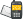It is currently 22 Mar 2019, 08:12### GMAT Club Daily Prep

#### Thank you for using the timer - this advanced tool can estimate your performance and suggest more practice questions. We have subscribed you to Daily Prep Questions via email.

Customized
for You

we will pick new questions that match your level based on your Timer History

Track

every week, we’ll send you an estimated GMAT score based on your performance

Practice
Pays

we will pick new questions that match your level based on your Timer History

#### Not interested in getting valuable practice questions and articles delivered to your email? No problem, unsubscribe here.# GRE math Challenge #43-If N = 3^x*5^y, where x and yQuestion banks Downloads My Bookmarks Reviews Important topics
Author Message
TAGS:
DirectorJoined: 16 May 2014
Posts: 595
GRE 1: Q165 V161Followers: 94

Kudos [?]: 456 , given: 64

GRE math Challenge #43-If N = 3^x*5^y, where x and y [#permalink]
Expert's post00:00

Question Stats:100% (01:06) correct0% (00:00) wrongbased on 8 sessions
If $$N = 3^x*5^y$$, where x and y are positive integers, and N has 12 positive factors, what is the value of N?

 Column A Column B 9 is not a factor 125 is a factor

A. Only Column A is sufficient.
B. Only Column B is sufficient.
C. Column A and B both are needed
D. Column A and B both are insufficient
E. None of the columns are needed.
_________________

My GRE Resources
Free GRE resources | GRE Prep Club Quant Tests
If you find this post helpful, please press the kudos button to let me know !InternJoined: 26 Mar 2015
Posts: 1
Followers: 0

Kudos [?]: 0 , given: 0

Re: GRE math Challenge #43 [#permalink]
That is not a GRE question, just FYI.

Posted from my mobile deviceDirectorJoined: 16 May 2014
Posts: 595
GRE 1: Q165 V161Followers: 94

Kudos [?]: 456 , given: 64

Re: GRE math Challenge #43 [#permalink]
Expert's post
Yes you are right!
The idea is to solve maths problems regardless what exam it is. Just to challenge brains._________________

My GRE Resources
Free GRE resources | GRE Prep Club Quant Tests
If you find this post helpful, please press the kudos button to let me know !ManagerJoined: 27 Sep 2017
Posts: 112
Followers: 1

Kudos [?]: 30 , given: 4

Re: GRE math Challenge #43-If N = 3^x*5^y, where x and y [#permalink]
soumya1989 wrote:
If $$N = 3^x*5^y$$, where x and y are positive integers, and N has 12 positive factors, what is the value of N?

 Column A Column B 9 is not a factor 125 is a factor

A. Only Column A is sufficient.
B. Only Column B is sufficient.
C. Column A and B both are needed
D. Column A and B both are insufficient
E. None of the columns are needed.

For me, the ans would be A, we are told that $$N = 3^x*5^y$$, where x and y are positive integers, and N has 12 positive factors.

then (x+1)(y+1)=12. Let us do some prime factorization of 12.

12=2*6=1*12=3*4 We should rule out the pair 1*12 since x+1 or y+1=1, then x or y=0. Yet, we are told that x and y are positive integers. Now for cloumn A, we are told that 9 is not a factor of N. This leads us to know x=1 and y=5. then $$N = 3^1*5^5$$.

For column B, we are told that 125 is a factor. That means that y>=3. So, y would be 3 or 5. B can't help us decide the value of N.

ANS:ARe: GRE math Challenge #43-If N = 3^x*5^y, where x and y   [#permalink] 11 Mar 2018, 05:38
Display posts from previous: Sort by

# GRE math Challenge #43-If N = 3^x*5^y, where x and yQuestion banks Downloads My Bookmarks Reviews Important topicsPowered by phpBB © phpBB Group Kindly note that the GRE® test is a registered trademark of the Educational Testing Service®, and this site has neither been reviewed nor endorsed by ETS®.